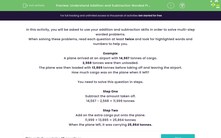# Solve Worded Addition and Subtraction Problems

In this worksheet, students will be asked to use addition and subtraction skills in order to solve multi-step worded problems.Key stage:  KS 2

Curriculum topic:   Number: Addition and Subtraction

Curriculum subtopic:   Solve Multi-Step Add/Subtract Problems

Difficulty level:#### Worksheet Overview

In this activity, you will be asked to use your addition and subtraction skills in order to solve multi-step worded problems.

When solving these problems, read each question at least twice and look for highlighted words and numbers to help you.

Example

A plane arrived at an airport with 14,567 tonnes of cargo.

The plane was then loaded with 13,865 tonnes before taking off and leaving the airport.

How much cargo was on the plane when it left?You need to solve this question in steps.

Step One

Subtract the amount taken off.

14,567 - 2,568 = 11,999 tonnes

Step Two

Add on the extra cargo put onto the plane.

11,999 + 13,865 = 25,864 tonnes

When the plane left, it was carrying 25,864 tonnes.

Let's have a go at some questions now.

### What is EdPlace?

We're your National Curriculum aligned online education content provider helping each child succeed in English, maths and science from year 1 to GCSE. With an EdPlace account you’ll be able to track and measure progress, helping each child achieve their best. We build confidence and attainment by personalising each child’s learning at a level that suits them.

Get started